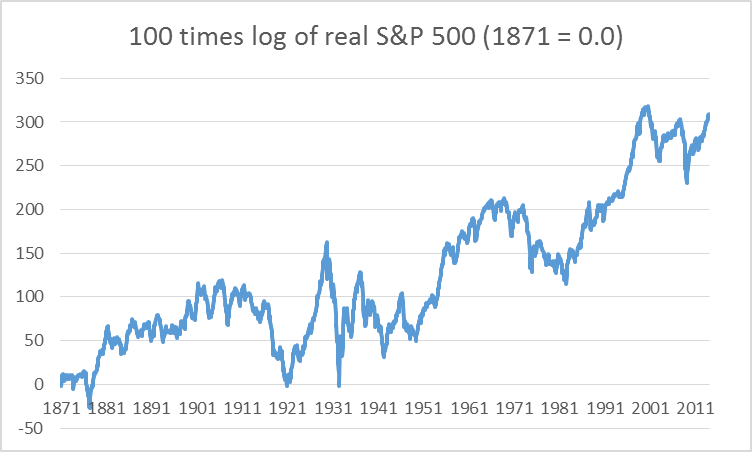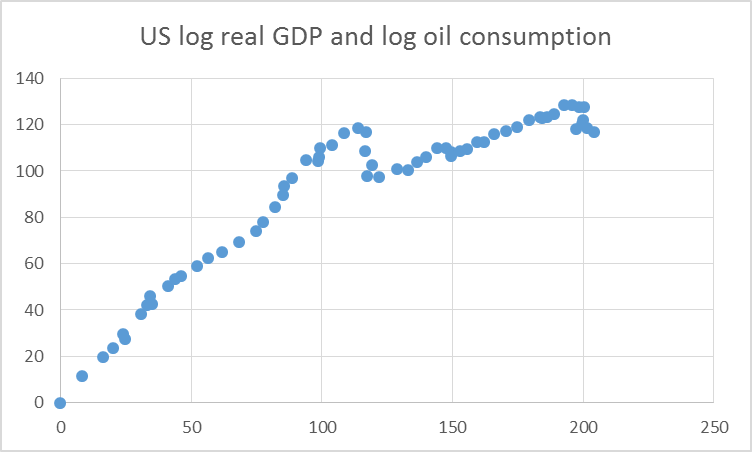Use of Logarithms in Economics

Why do economists always want to take the natural logarithm of everything? Here’s the answer, if you don’t mind looking at a few equations and graphs.

Let me begin with a quick review of compound interest rates. If you invest an amount$P_{1}$ for one year at an r% interest rate, at the end of the year you’ll have an amount$P_{2}=(1+r)P_{1}$. For example, with a 4% return,$P_{2}=1.04P_{1}.$ Sometimes your interest might be compounded, for example, you get your money back plus 2% at the end of six months, and then can earn 2% interest on both your original principal as well as on the first six month’s interest:$P_{2}=(1+r/2)(1+r/2)P_{1}=(1+r/2)^{2}P_{1}$. With an interest rate of r = 4%, compounded twice annually like this your money would actually have grown 4.04% at the end of the year. Compounded quarterly,$P_{2}=(1+r/4)^{4}P_{1},$ or about 4.06% at the end of the year. It turns out the formula$(1+r/n)^{n}$ converges to a particular function as the frequency of compounding n gets arbitrarily large, this limit being$\underset{n\rightarrow \infty }{\lim }(1+r/n)^{n}=e^{r},$ where e is a special number (approximately equal to 2.72) that possesses this and a number of other amazing properties. Thus if you earn r% interest that is compounded continuously, at the end of the year your money will have grown by$P_{2}/P_{1}=e^{r}$. For example, if r = 4%, continuous compounding would actually give you 4.08% at the end of the year, a little more than the 4.06% from quarterly compounding or 4.0% with no compounding.

Taking natural logarithms is just the inverse of the above operation:$ln(P_{2}/P_{1})=r$, or since the log of a ratio is the difference of the logs,$ln(P_{2})-ln(P_{1})=r.$ In other words, taking the difference between the log of a stock price in year 2 and the log of the price in year 1 is just calculating a rate of return on the holding, quoted in terms of a continuously compounded rate.

For low values of r, the continuously compounded return is almost the same as the noncompounded return, so that the log difference is almost the same number as the percentage change. For the example just given, the percentage change is$(P_{2}-P_{1})/P_{1}=0.0408$ whereas the log change is$ln(P_{2})-ln(P_{1})=0.04.$ So any time that you see a graph that is measured in logs, an increase of 0.01 on that scale corresponds very closely to a 1% increase. A graph that is a straight line over time when plotted in logs corresponds to growth at a constant percentage rate each year.

Using logs, or summarizing changes in terms of continuous compounding, has a number of advantages over looking at simple percent changes. For example, if your portfolio goes up by 50% (say from $100 to$150) and then declines by 50% (say from $150 to$75), you’re not back where you started. If you calculate your average percentage return (in this case, 0%), that’s not a particularly useful summary of the fact that you actually ended up 25% below where you started. By contrast, if your portfolio goes up in logarithmic terms by 0.5, and then falls in logarithmic terms by 0.5, you are exactly back where you started. The average log return on your portfolio is exactly the same number as the change in log price between the time you bought it and the time you sold it, divided by the number of years that you held it.

Logarithms are often a much more useful way to look at economic data. For example, here is a graph of an overall U.S. stock price index going back to 1871. Plotted on this scale, one can see nothing in the first century, whereas the most recent decade appears insanely volatile.

On the other hand, if you plot these same data on a log scale, a vertical move of 0.01 corresponds to a 1% change at any point in the figure. Plotted this way, it’s clear that, in percentage terms, the recent volatility of stock prices is actually modest relative to what happened in the Great Depression in the 1930′s.

There are a couple of other changes that can make the graph even more meaningful and easy to read. For one thing, we probably want to take out the effect of inflation. The graph below plots the natural log of the real instead of the nominal stock price, and subtracts off the log from 1871, so that the graph starts at zero and reports cumulative log returns since then. The numbers have also been multiplied by 100, so that if the value goes up by 1 unit on this graph, it corresponds to a 1% increase in the real value of stocks.100 times difference between natural log of real stock price index at date t minus log of real stock price in 1871:M1.

Natural logs are also very convenient for describing relations between economic variables. For example, here’s a scatter plot of the relation between the log of U.S. real GDP on the horizontal axis (multiplied by 100 and normalized to begin at 0) and the log of U.S. oil consumption on the vertical axis. The relation between these series follows a straight line with slope of 1 up until about 1970, corresponding to an income elasticity of oil demand of around 1– for every 1% increase in real GDP, U.S. oil consumption also rose by 1%. By contrast, since 1970, the slope of the graph is about 0.2– U.S. real GDP has grown at a (continuously compounded) annual rate since then of 2.9%, whereas oil consumption has only grown 0.6%. You can read those numbers directly off the graph below by taking the difference between any two points and dividing by the number of years separating them.Horizontal axis: 100 times difference between natural log of real GDP in year t and value in 1949. Vertical axis: 100 times difference between natural log of U.S. oil consumption in year t and 1949. Data set covers 1949 through 2012.

So next time somebody wants to summarize data in terms of natural logs, don’t complain– it’s usually a much more meaningful and robust way to see what’s going on.

This piece is cross-posted from Econbrowser with permission.